Class 8 RD Sharma Solutions – Chapter 3 Squares and Square Roots – Exercise 3.8

• Last Updated : 28 Dec, 2020

i) 5

Solution: We will use Long Division Method to find square root of 5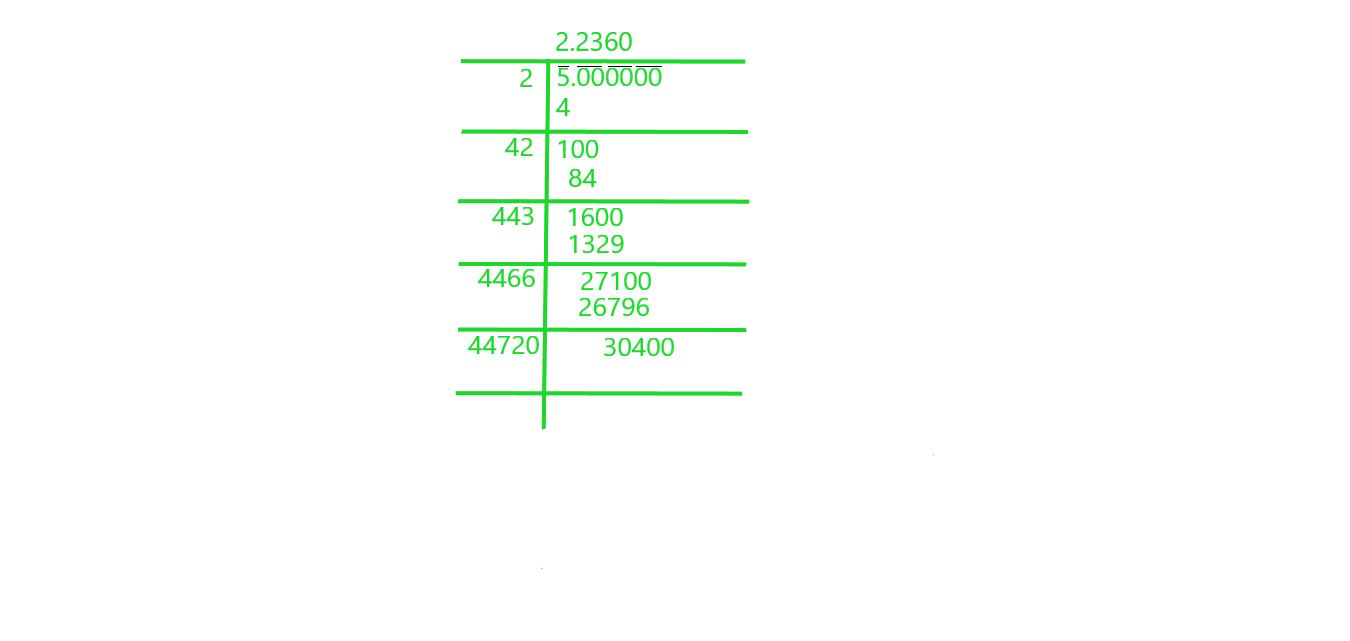Square root of 5 is 2.2360

ii) 7

Solution: We will use Long Division Method to find square root of 7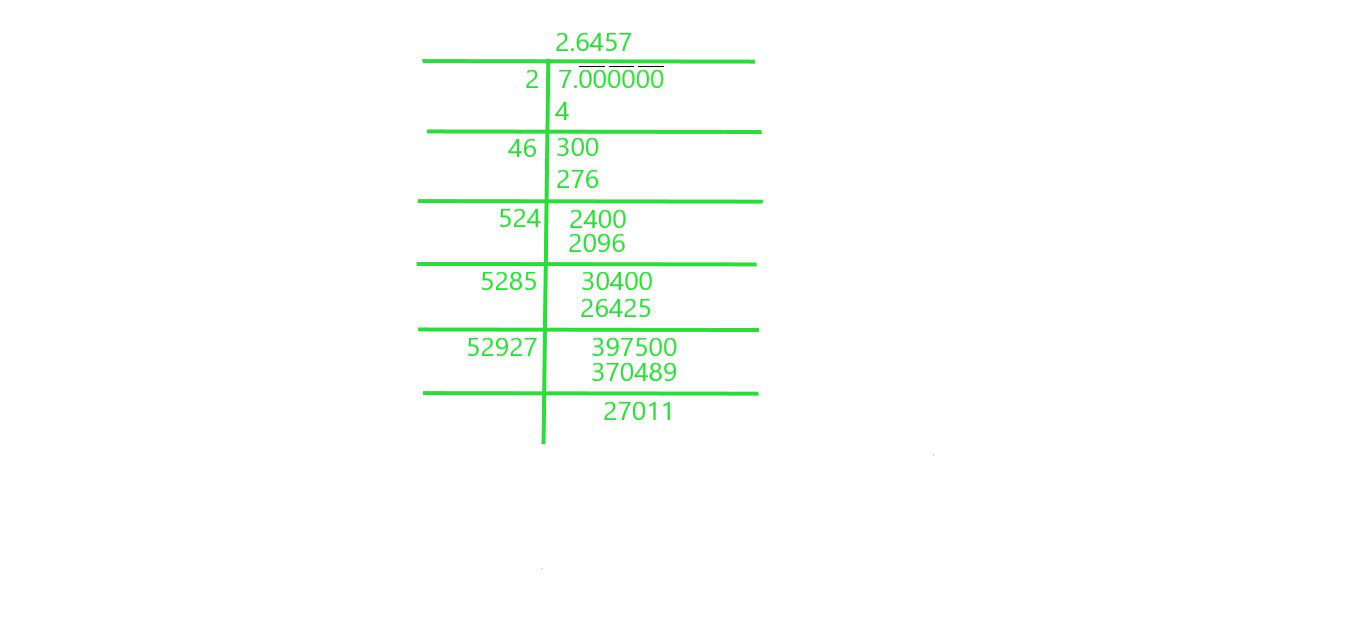Square root of 7 is 2.6457

iii) 17

Solution: We will use Long Division Method to find square root of 17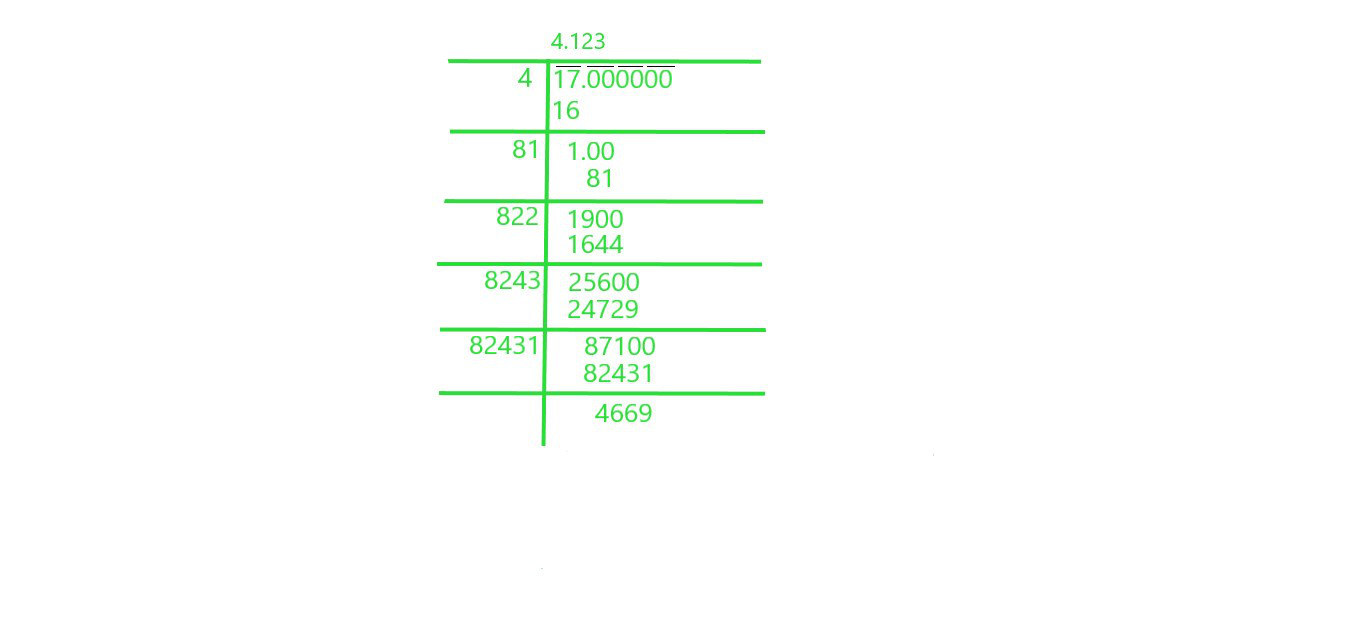Square root of 17 is 4.123

iv) 20

Solution: We will use Long Division Method to find square root of 20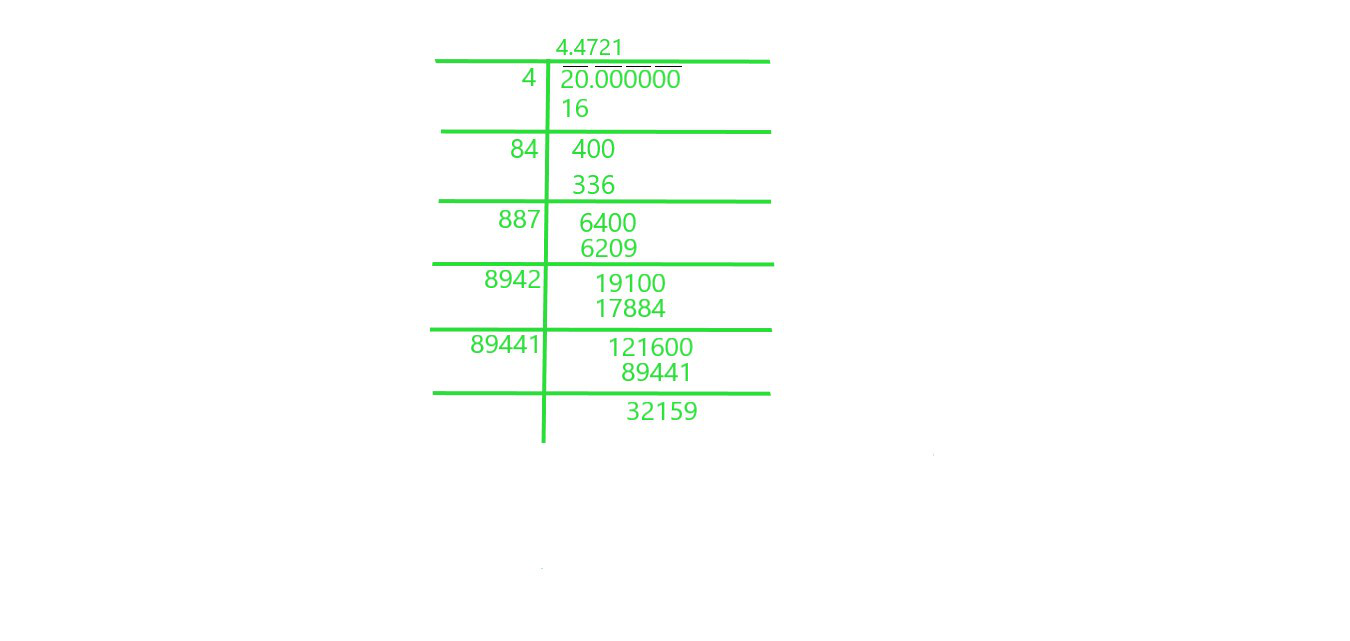Square root of 20 is 4.4721

v) 66

Solution: We will use Long Division Method to find square root of 66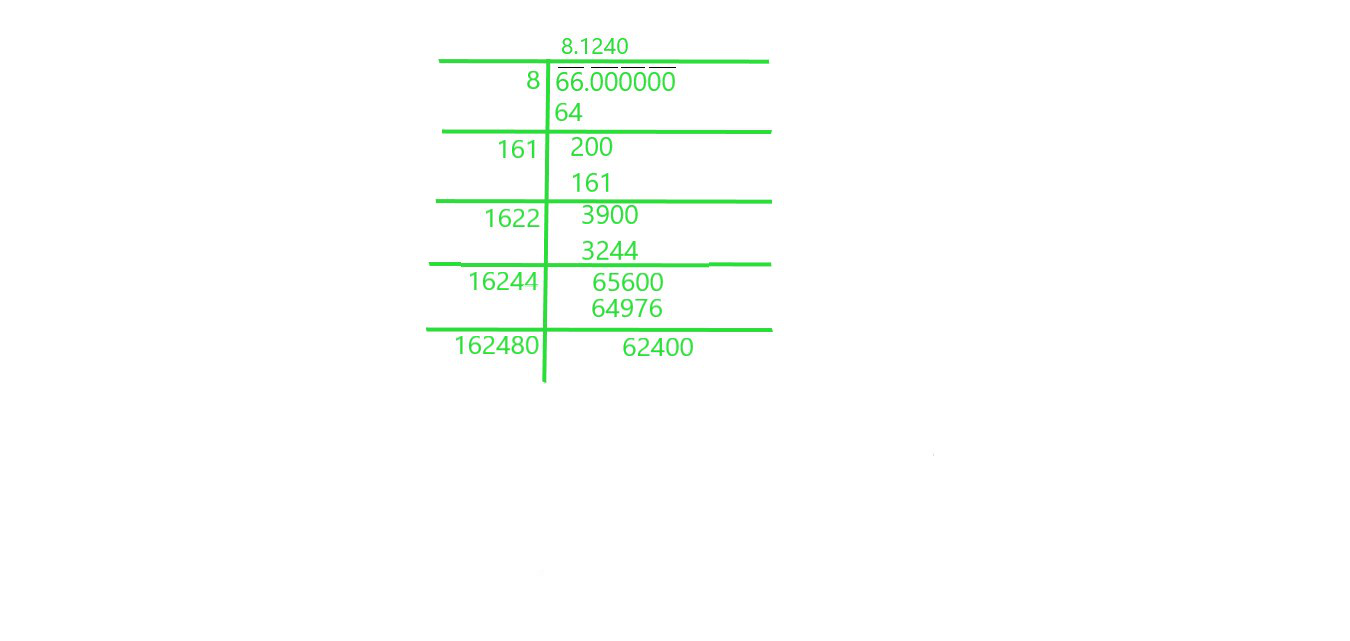Square root of 66 is 8.1240

vi) 427

Solution: We will use Long Division Method to find square root 427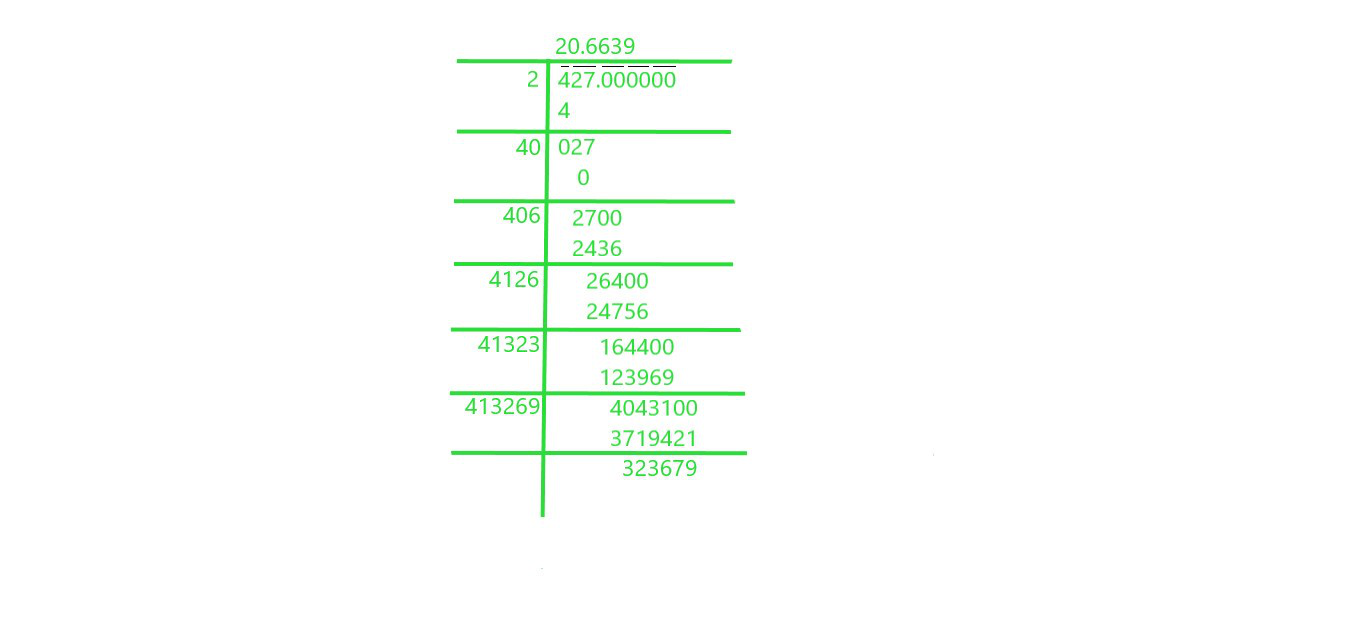Square root of 427 is 20.6639

vii) 1.7

Solution: We will use Long Division Method to find square root of 1.7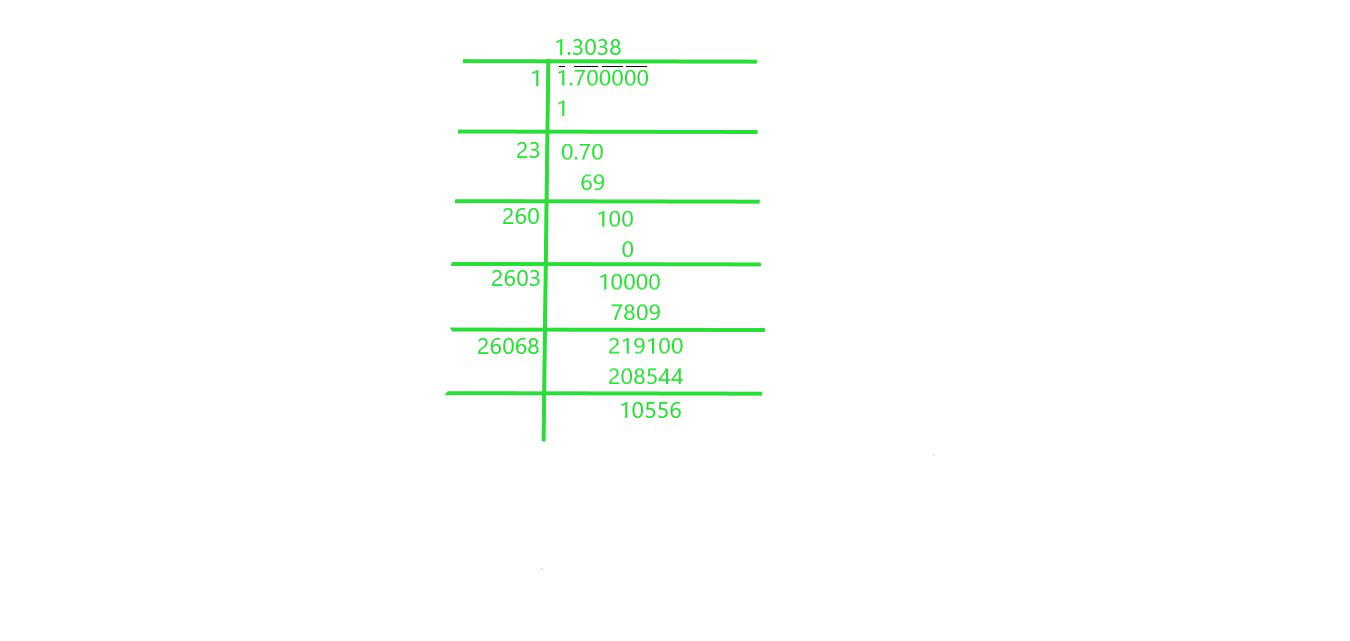Square root of 1.7 is 1.3038

viii) 23.1

Solution: We will use Long Division Method to find square root of 23.1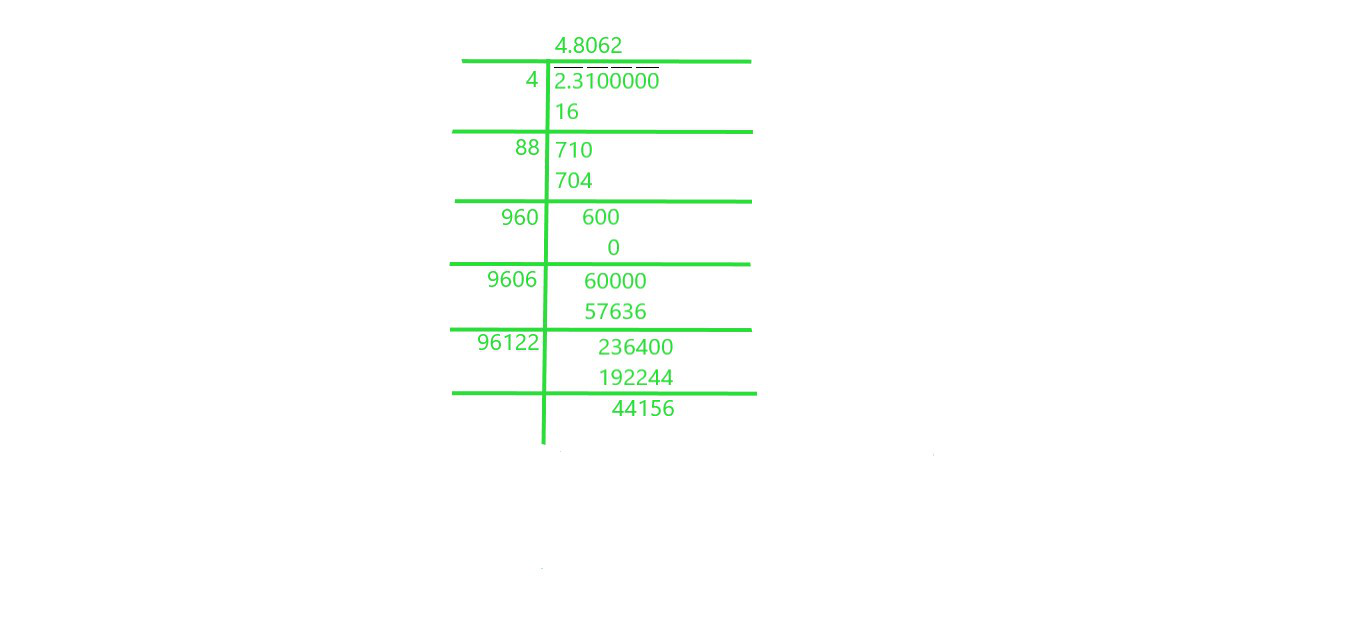Square root of 23.1 is 4.8062

ix) 2.5

Solution: We will use Long Division Method to find square root of 2.5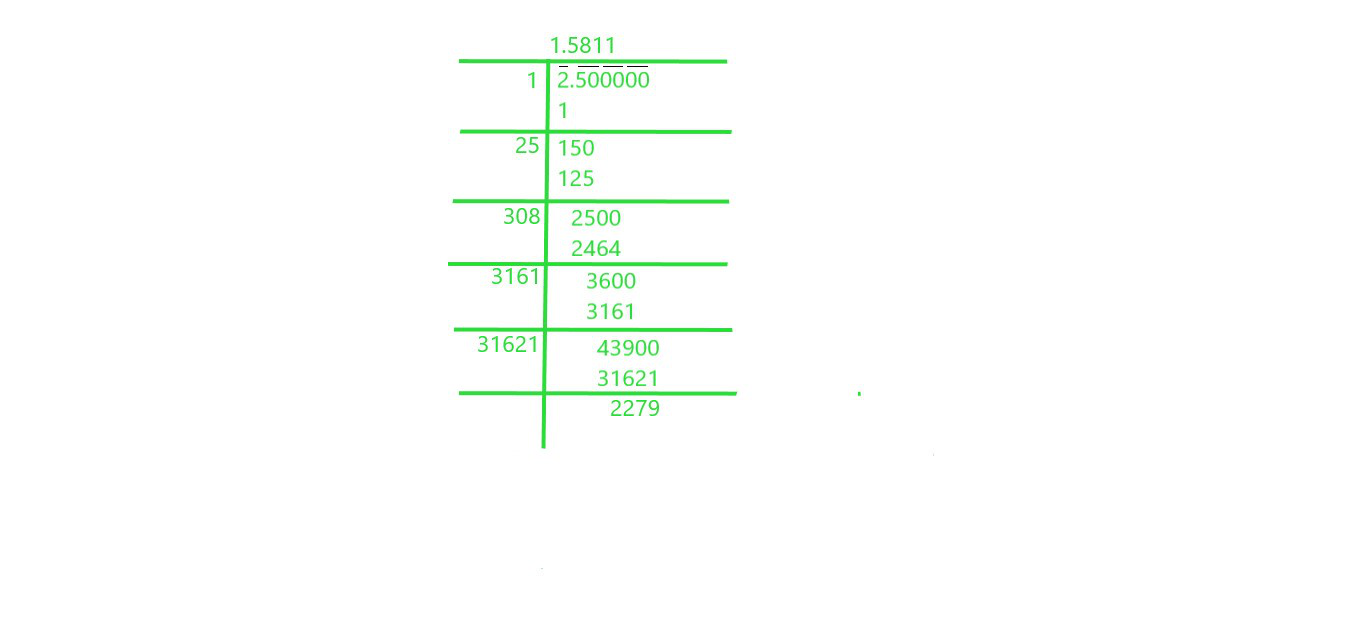Square root of 2.5 is 1.5811

x) 237.615

Solution: We will use Long Division Method to find square root of 237.615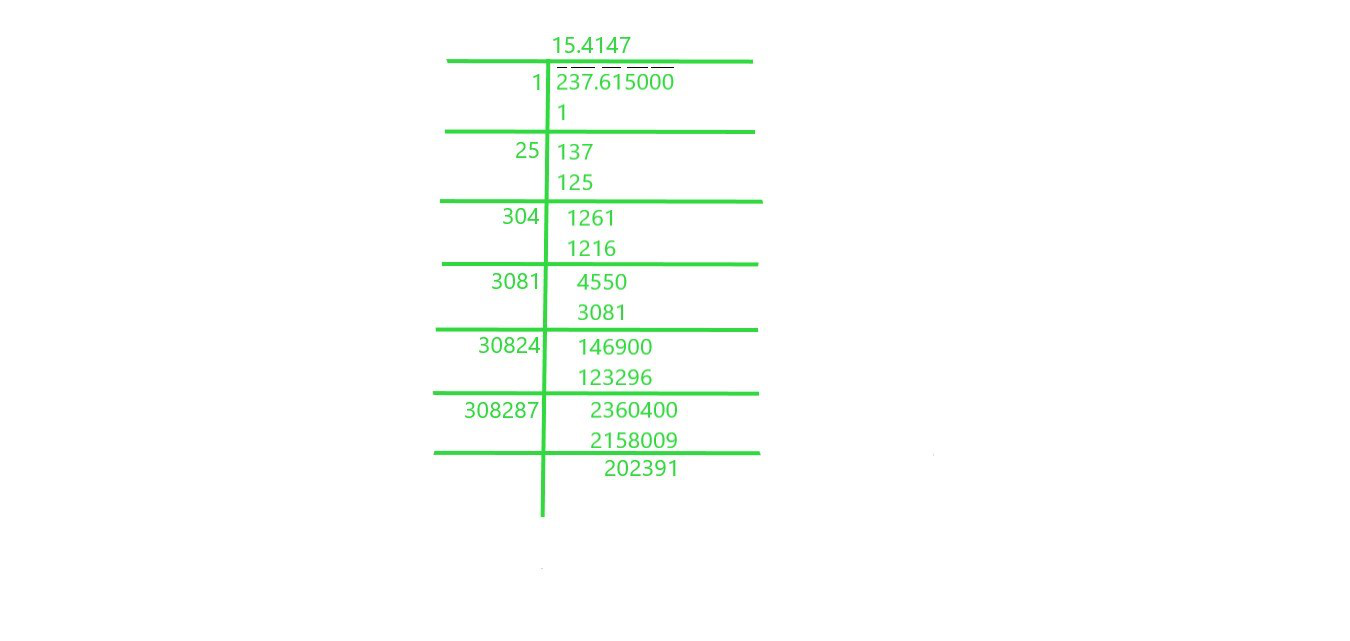Square root of 237.615 is 15.4147

xi) 15.3215

Solution: We will use Long Division Method to find square root of 15.3215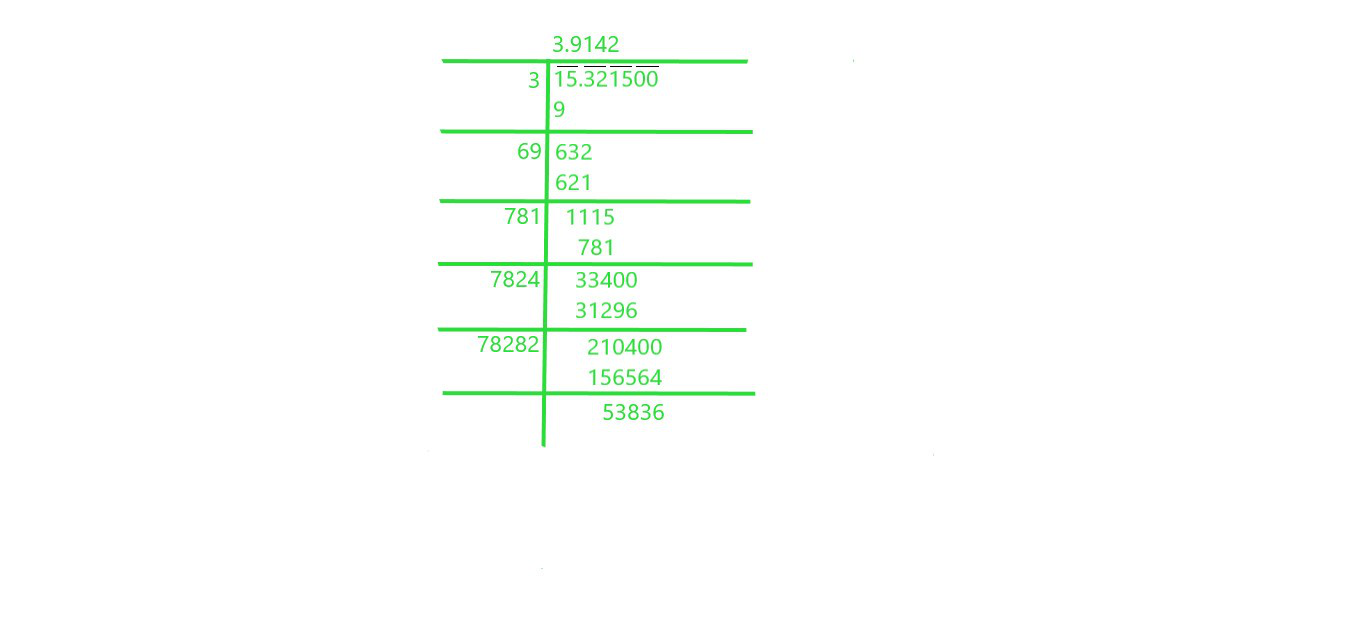Square root of 15.3215 is 3.9142

xii) 0.9

Solution: We will use Long Division Method to find square root of 0.9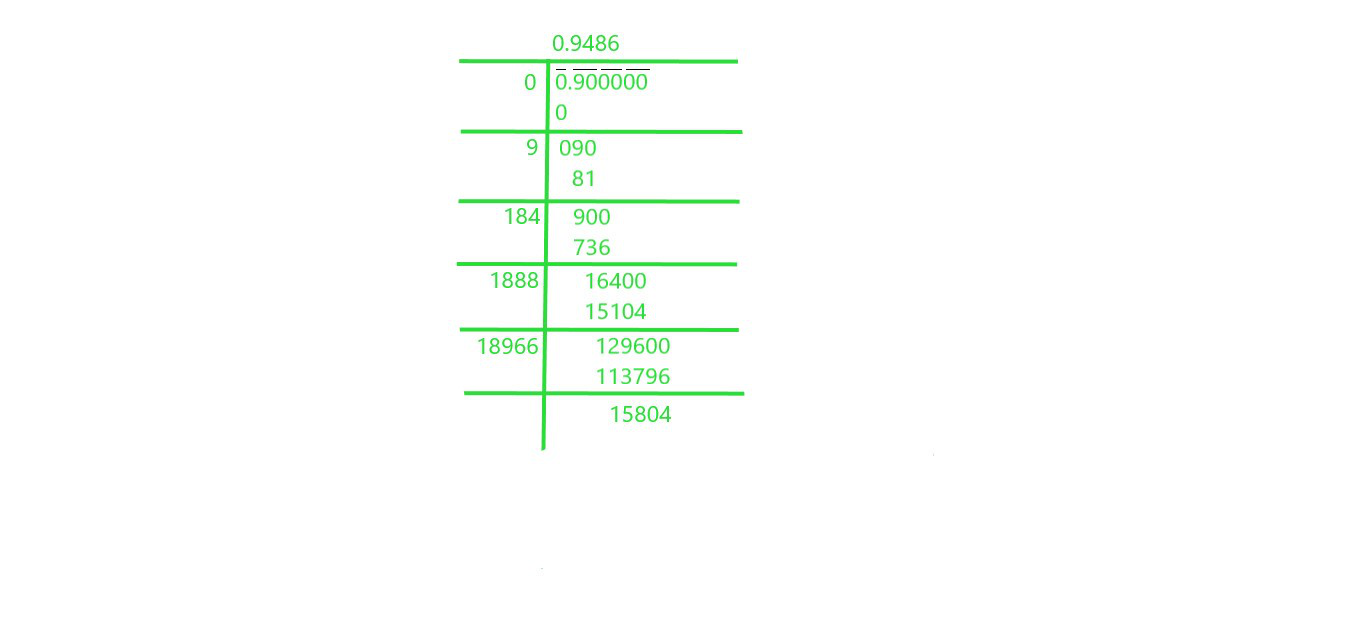Square root of 0.9 is 0.9486

xiii) 0.1

Solution: We will use Long Division Method to find square root of 0.1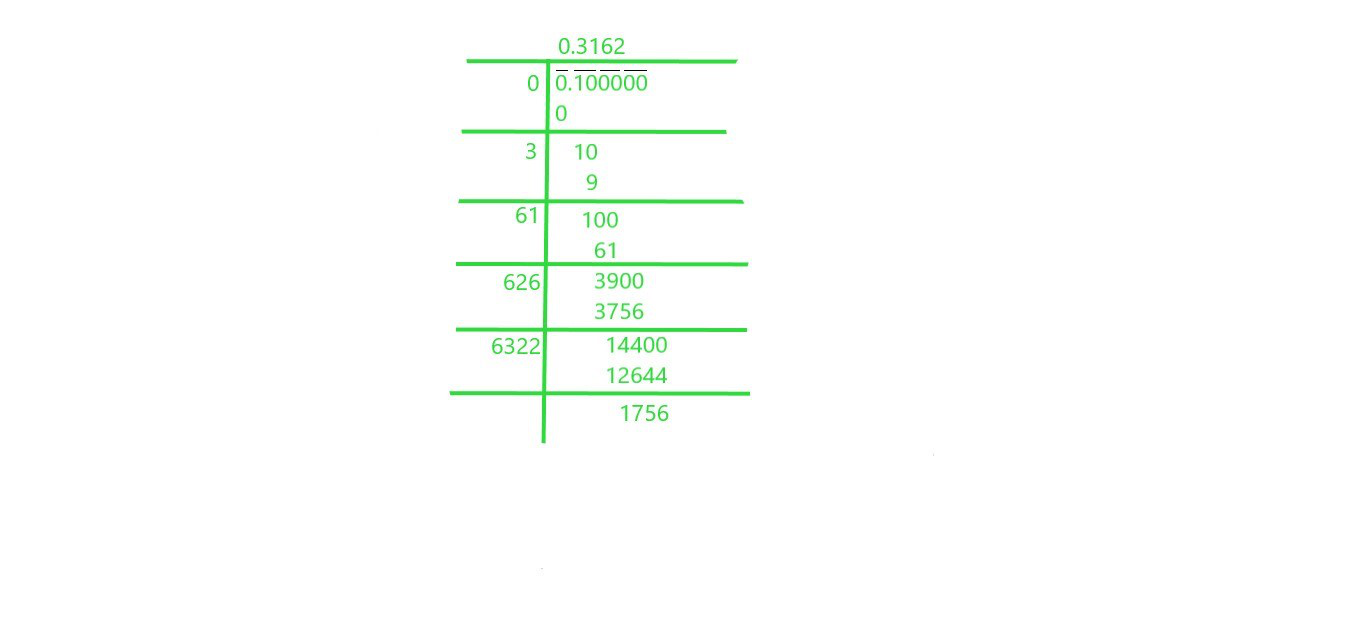Square root of 0.1 is 0.3162

xiv) 0.016

Solution: We will use Long Division Method to find square root of 0.016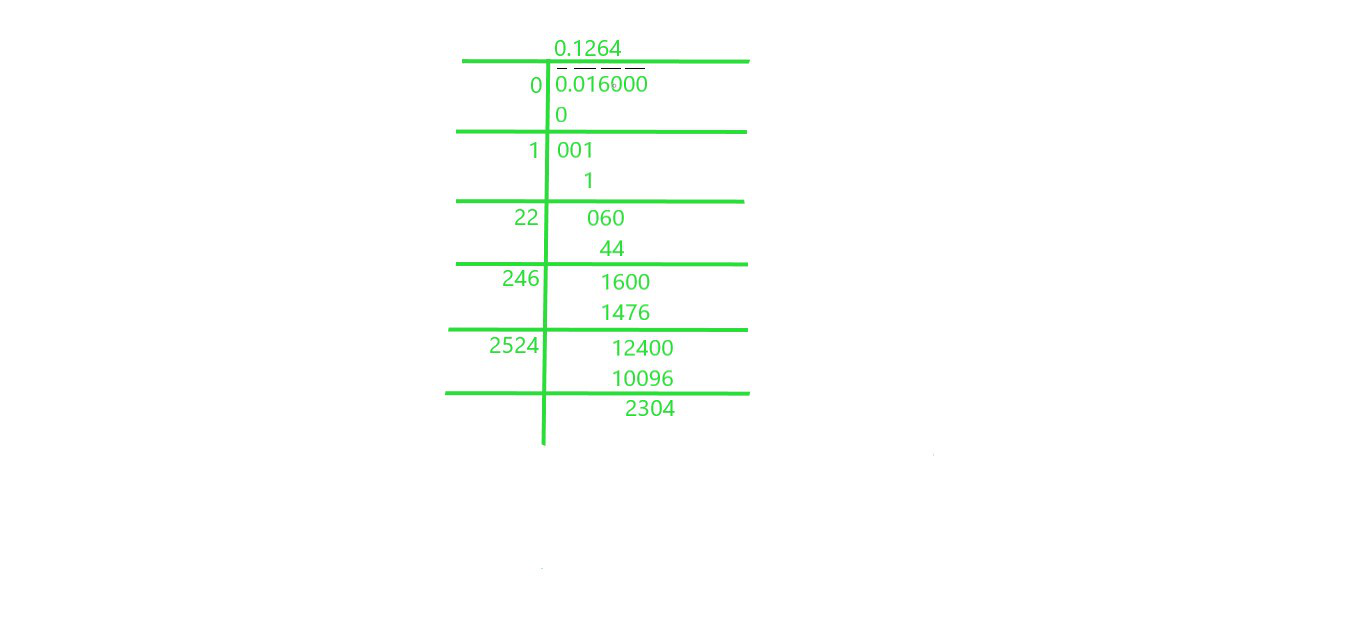Square root of 0.016 is 0.1264

xv) 0.00064

Solution: We will use Long Division Method to find square root of 0.00064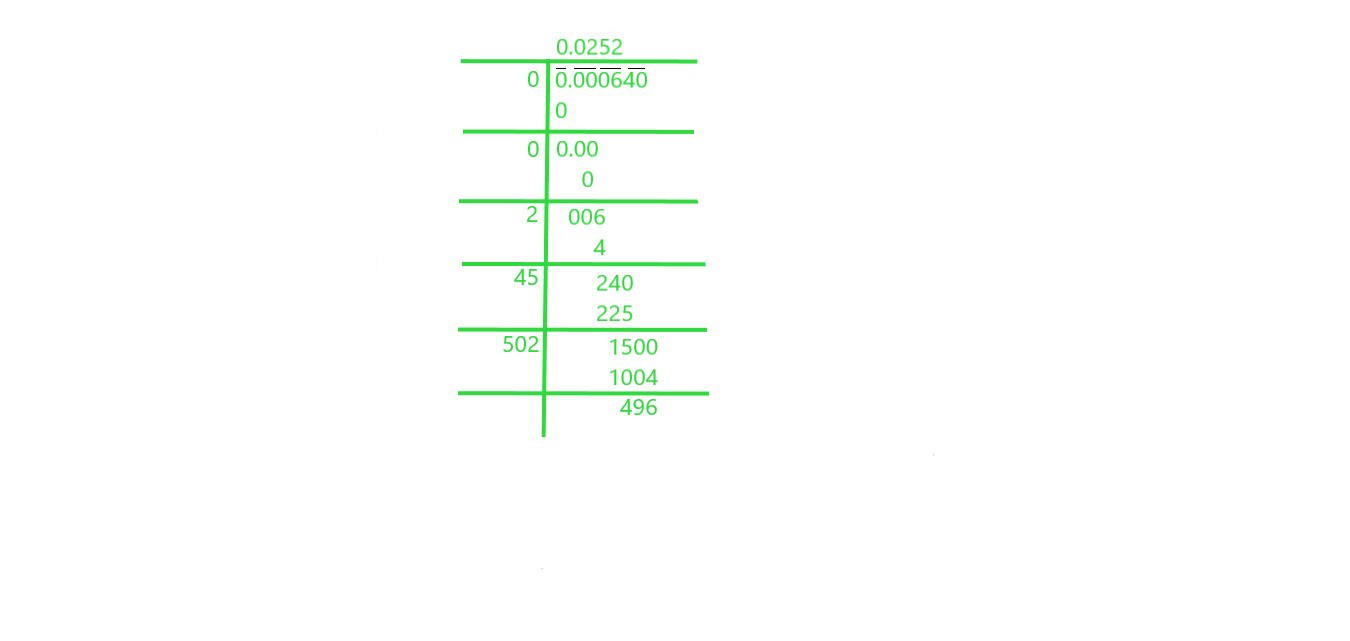Square root of 0.00064 is 0.0252

xvi) 0.019

Solution: We will use Long Division Method to find square root of 0.019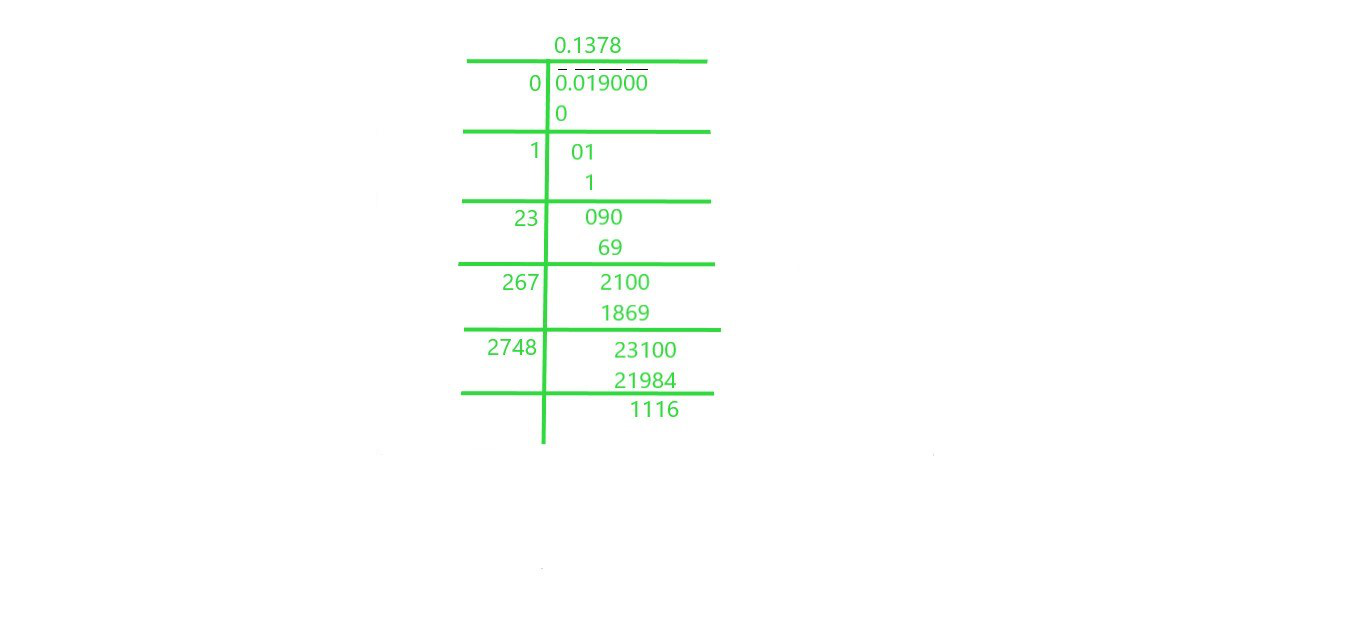Square root of 0.019 is 0.1378

xvii) 7/8

Solution:  We will use Long Division Method to find square root of 7/8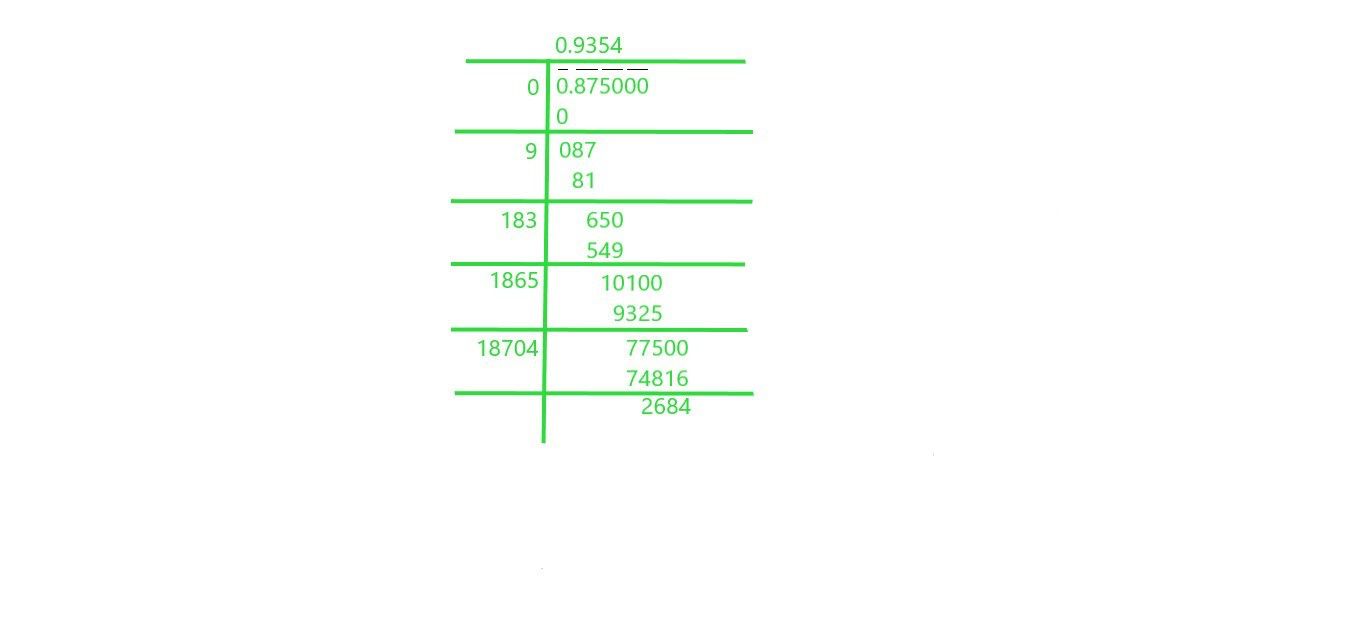Square root of 7/8 is 0.9354

xviii) 5/12

Solution: We will use Long Division Method to find square root of 5/12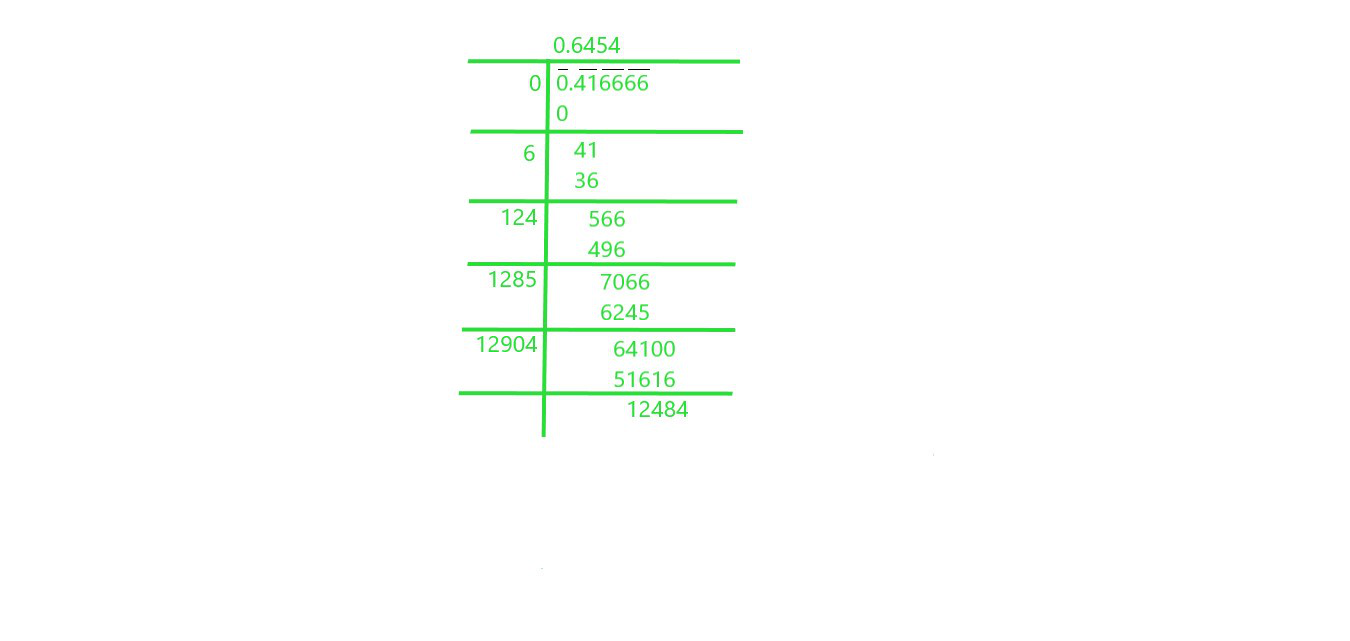Square root of 5/12 is 0.6454

xix) 2 ½

Solution: We will use Long Division Method to find square root of 2 ½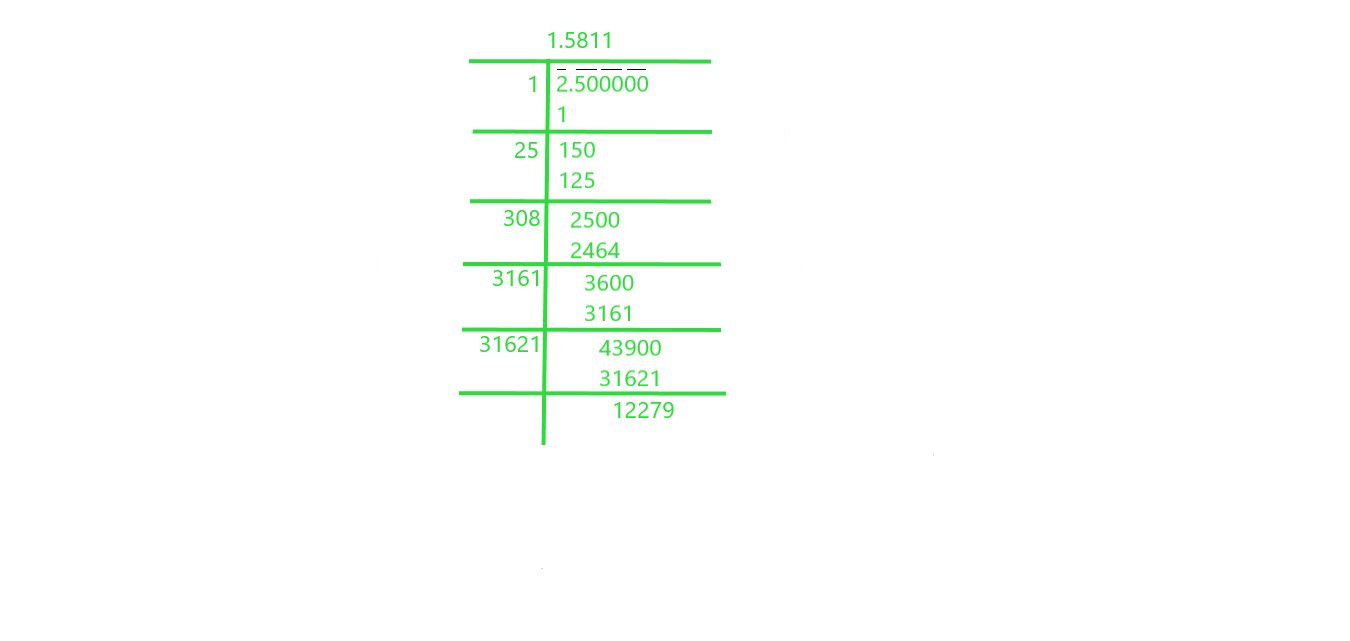Square root of 2 ½ is 1.5811

xx) 287 5⁄8

Solution: We will use Long Division Method to find square root of 287 5⁄8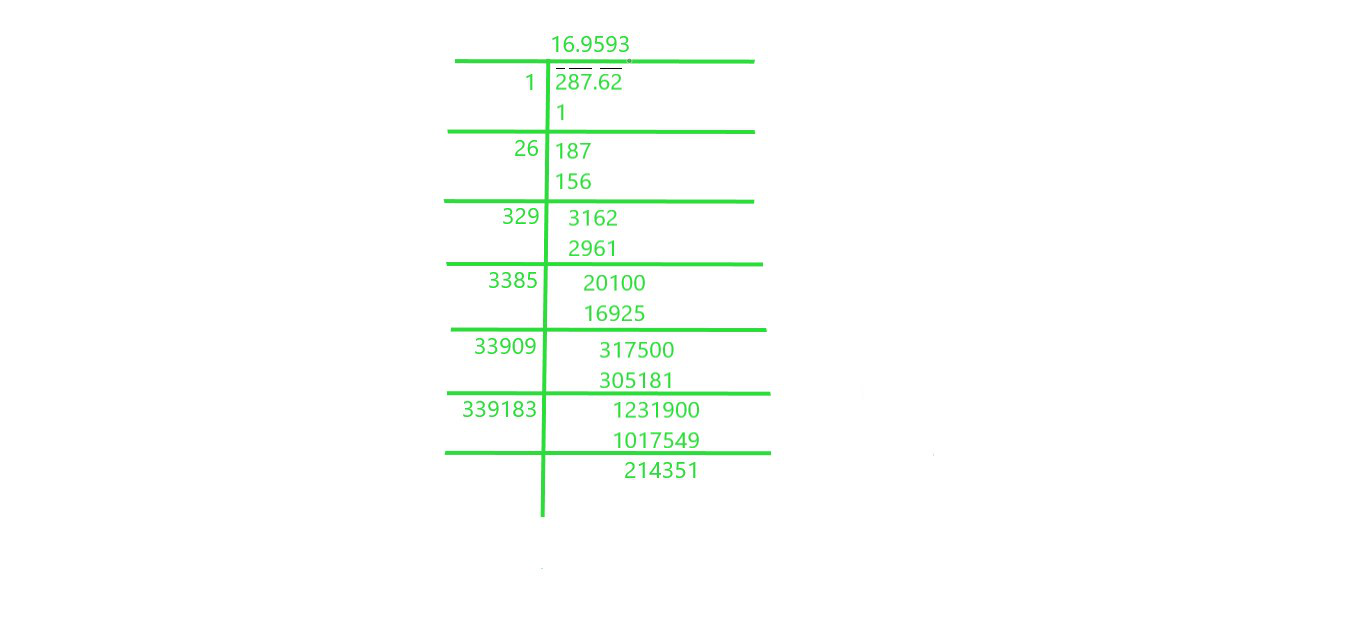Square root of 287 5⁄8 is 16.9593

Question 2. Find the square root of 12.0068 correct to four decimal places.

Solution: We will use Long Division Method to find square root of 12.0068 correct to four decimal place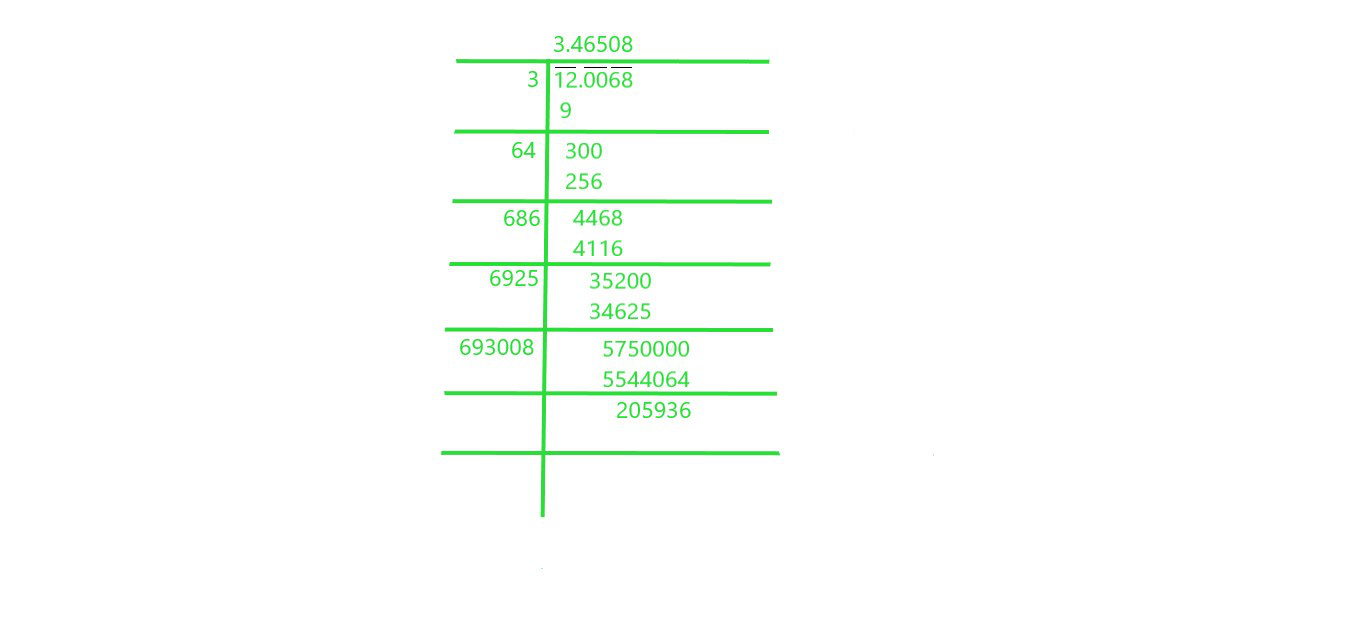The square root of 12.0068 correct to four decimal place is 3.46508

Question 3. Find the square root of 11 correct to five decimal places.

Solution: We will use Long Division Method to find square root of 11 up to five decimal place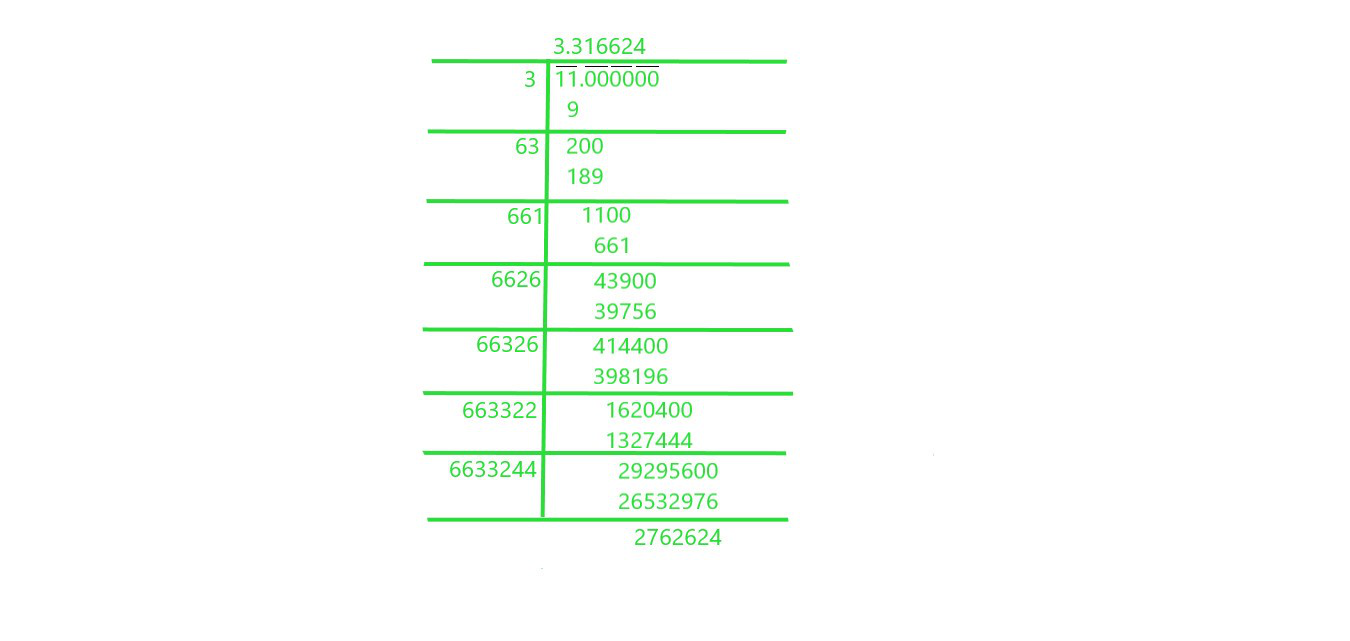The square root of 11 correct to five decimal place is 3.316624

Question 4. Give that: √2 = 1.414, √3 = 1.732, √5 = 2.236 and √7 = 2.646, Evaluate each of the following :

(i) √(144/7)    (ii) √(2500/3)

Solution:

(i) √(144/7)

We can write √144 as √12×12 and we will calculate square root of √7

= 2.646 = √(12×12)/ √7

= 12/ 2.646 = 4.535

(ii) √(2500/3)

We will find factor of √2500 = √2x2x5x5x5x5, which is equal to 50 and we will calculate square root of √3

which is equal to 1.732 = 50/1.732 = 28.867

Question 5. Given that √2 = 1.414, √3 = 1.732, √5 = 2.236 and √7 = 2.646 find the square roots of the following :

(i) 196/75     (ii) 400/63     (iii) 150/7     (iv) 256/5    (v) 27/50

Solution:

(i) 196/75

We have to calculate square root of √(196/75) , we can write it as √(196) / √(75)

Now find the factors of both numerator and denominator, we can write it as:

= √(14×14) / √(3x5x5) = 14/5√3

5√3 is equal to 5×1.732

= 8.66 = 1.617

(ii) 400/63

We have to calculate square root of √(400/63), we can write it as √(400)/√(63)

Now find the factors of both numerator and denominator, we can write it as

= √(20×20)/√(3x3x7)

= 20 / 3√7

3√7 is equal to 3×2.646

= 7.938

= 2.520

(iii) 150/7

We have to calculate square root of √(150/7), we can write it as √(150) / √(7)

Now find the factors of both numerator and denominator, we can write it as:

= √(3x5x5x2)/√(7) = 5x√3x√2 / √7

5x√3x√2 is equal to 5 x 1.732 x 1.414

= 12.245

= 12.245 / 2.646

= 4.628

(iv) 256/5

We have to calculate square root of √(256/5), we can write it as √(256) / √(5)

Now find the factors of both numerator and denominator, we can write it as:

= √(16×16) / √(5)

= 16 / √5

√5 is equal to 2.236

= 7.155

(v) 27/50

We have to calculate square root of √(27/50), we can write it as √(27) / √(50)

Now find the factors of both numerator and denominator, we can write it as:

= √(3x3x3) / √(2x5x5) = 3√3 / 5√2

3√3 is equal to 5.196 and 5√2 = 7.07

= 0.735

My Personal Notes arrow_drop_up